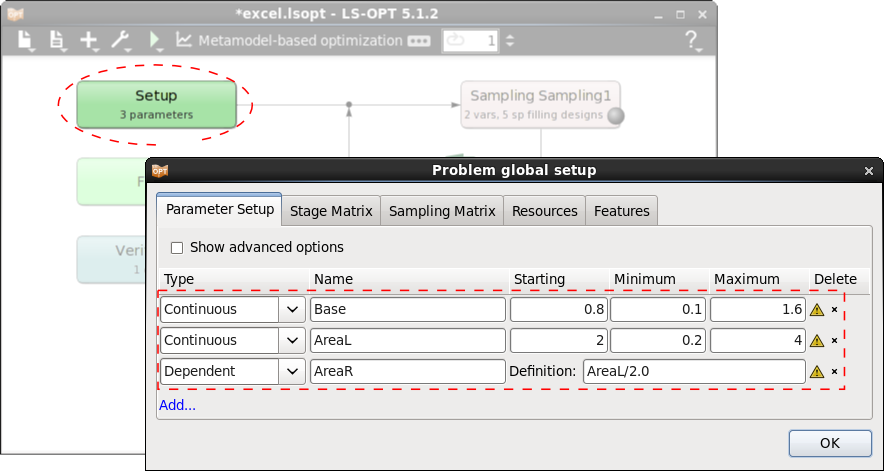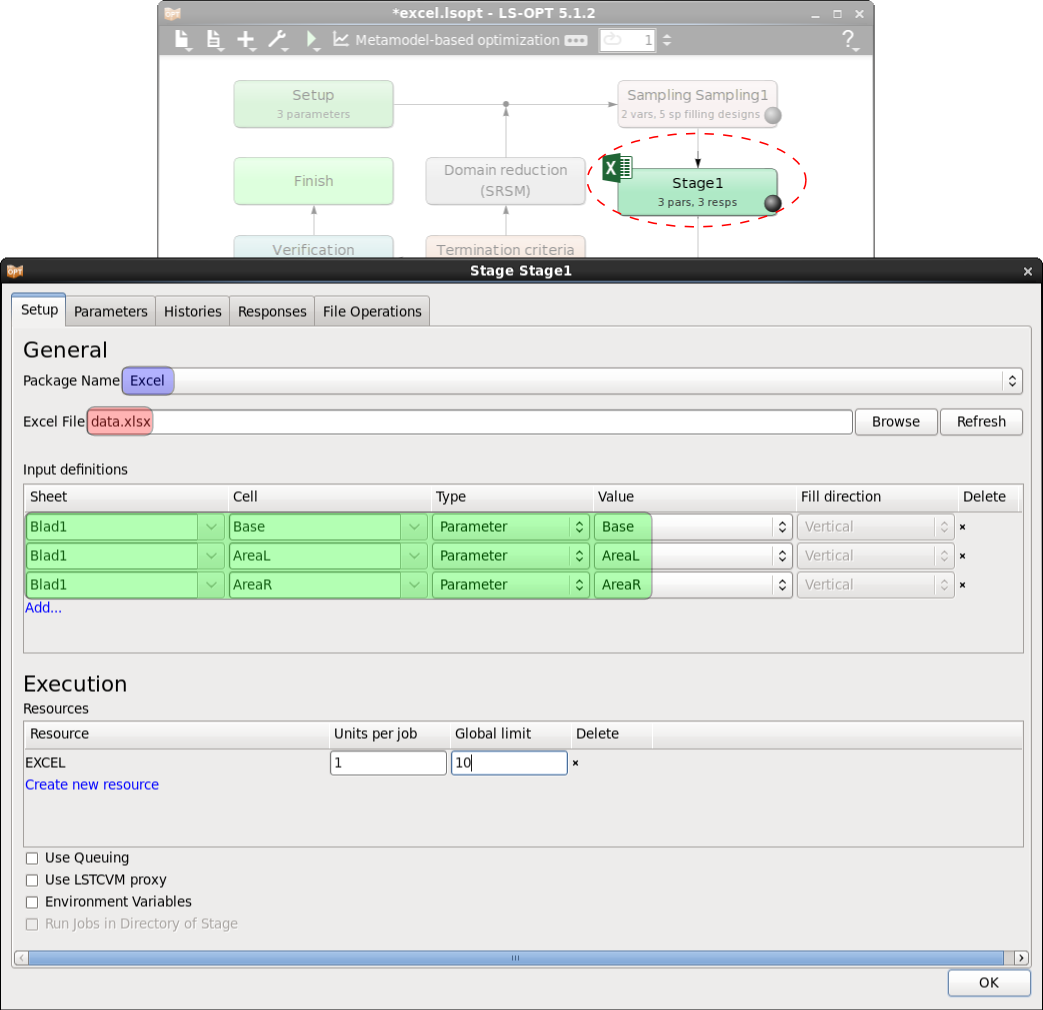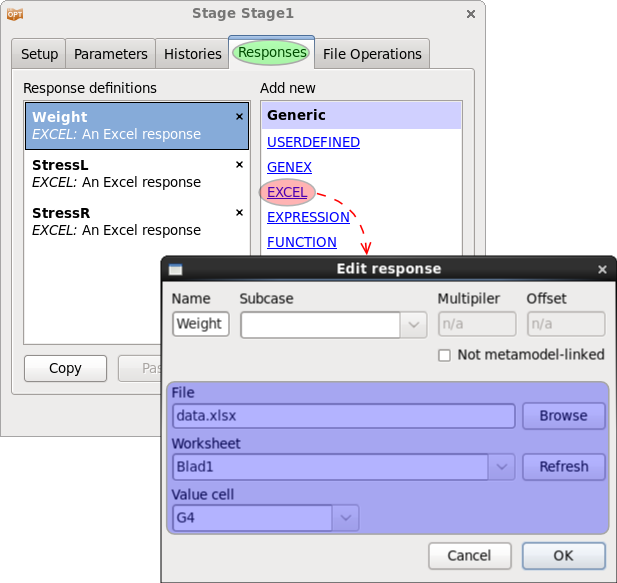x

# Integrating Excel

Integrating Excel in LS-OPT

Excel can be used as a solver or a post-processor in LS-OPT. It is possible to define parameters in an Excel sheet and extract responses or histories.Figure-1: The two-bar truss example and the Excel interface. Excel calculates the weight and the stresses depending on the cross-sectional areas of the bars and the distance between the supported nodes.

#### Define Design Parameter

Since the Excel input file is not a text file, the standard way to parameterize the input files cannot be used here. Before integrating Excel into LS-OPT, we first need to define the design parameters in Setup dialog as shown in the Figure-2 below.  Use the Add... button and enter type, name, and the other required definitions for each parameter. Excel input defintions will be used in the Excel stage defintion to couple the paramaters to Excel cells, see below.Figure-2: Parameter setup dialog. The parameters used in Excel need to be added manually.

#### Integrating Excel

Using the Excel interface can be seen as being similar to any other solver, with the main differences lying in its parameterization and in the  response and history definitions. Because the results need to be computed for several samples within an LS-OPT task, the Excel input file needs to be parameterized. This is achieved using Input definitions specified in the Stage dialog itself. These inputs may correspond to a single Excel cell or a group of cells in the input file, and are substituted for each sample (Figure-3 below).

The attributes used for Input definitions are Sheet, Cell, Type, Value and Fill Direction. For more details see LS-OPT Manual, 5.3.10 Excel.Figure-3: Stage setup dialog.

#### Define History / Response

The histories and responses to be extracted from an Excel sheet can be defined using EXCEL interface listed under Generic history and responses interfaces. The cells and/or arrays of cells of an Excel document can be defined as LS-OPT histories or responses.

A response definition using Excel is presented in the Figure-4 below.Figure-4: Definition of an Excel response.

An example is provided for download below. Excel is used to solve the two-bar truss problem, Figure-1. The same example is solved in the LS-OPT Manual, 18.1 Two-bar truss using expressions.